## 20 Questions MCQ Test Math Olympiad for Class 5 | Olympiad Test: Number System

Description
Attempt Olympiad Test: Number System | 20 questions in 40 minutes | Mock test for Class 5 preparation | Free important questions MCQ to study Math Olympiad for Class 5 for Class 5 Exam | Download free PDF with solutions
QUESTION: 1

### What is the predecessor of the greatest six-digit number?

Solution:

The greatest six-digit number is 999999.
Therefore, predecessor of the greatest
six-digit number is
999999 – 1 = 999998.
Hence, the correct answer is C.

QUESTION: 2

### What is difference between the place values of two 5’s in the number 4598351?

Solution:

The given number is 45, 98, 351.
∴ Place value of 5 at Lakhs place in the given number = 5 × 100000 = 500000
Place value of 5 at Tens place in the given number = 5 × 10 = 50
∴ Difference between the place values of two 5’s in the given number = 500000 – 50 = 499950

Hence, the correct answer is A.

QUESTION: 3

###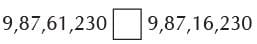Which of the following signs can be placed in the box between the two numbers?

Solution:

The given numbers are 9, 87, 61, 230 and 9, 87, 16, 230. Both of these numbers have 8 digits.

These two numbers can be written in
the place value table as follows: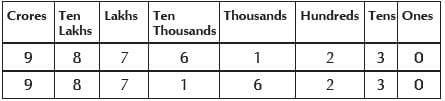Comparing the digits at ten thousands place, 6 > 1.

9, 87, 61, 230 > 9, 87, 16, 230.

Therefore, the sign “>” can be placed in the box.

Hence, the correct answer is A.

QUESTION: 4

10000001 is the successor of ______

Solution:

Successor of a number is the number that comes next to the given number and it can be obtained by adding 1 to the given number.

So, 10000001 is the successor of
10000000.

Hence, the correct answer is B.

QUESTION: 5

How many three-digit numbers can be formed with the digits 3, 0 and 7 without repetition?

Solution:

The three-digit numbers that can be formed with the digits 3, 0 and 7 can be listed as 307, 370, 703, 730.
Thus, 4 three-digit numbers can be formed with the given digits without repetition.

Hence, the correct answer is C.

QUESTION: 6

Which are the respective greatest and the smallest numbers amongst 321987, 319240, 321978 and 321970?

Solution:

The given numbers can be placed in the place value table as follows: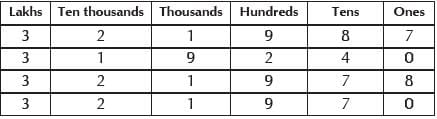It can be observed that the digits at lakhs place are the same.
On comparing the digits at ten thousands place, we get 2 > 1.
∴319240 is smallest amongst the given
numbers. Consider the numbers 321987, 321978 and 321970.
Here, the digits at thousands place are the same.
Also, the digits at hundreds place are the same. On comparing the digits at tens place, we get 8 > 7.
∴ 321987 > (321978, 321970)

Therefore, among the given numbers, 321987 and 319240 are the greatest and smallest numbers respectively

Hence, the correct answer is B.

QUESTION: 7

What is the difference between the greatest and smallest 5-digit numbers formed by using all the digits 3, 0, 9, 1 and 5?

Solution:

The given digits are 3, 0, 9, 1 and 5. The greatest 5-digit number formed by using all the given digits is 95310.
The smallest 5-digit number formed by using all the given digits is 10359.

∴ Required difference
= 95310 – 10359 = 84951

QUESTION: 8

Which of the following numbers is equal to 3 crore?

Solution:

It is known that 1 million =10 lakh and 1 crore = 100 lakh

∴ 1 crore = 10 × 10 lakh = 10 million

So, 3 crore = 3 × 10 million = 30 million

Therefore, 30 millions is equal to 3 crore.

Hence, the correct answer is B.

QUESTION: 9

How can the number fifty million twenty-one thousand two hundred thirty six be written using commas according to Indian system of numeration?

Solution:

Fifty million twenty-one thousand two hundred and thirty-six can be written in International system of numeration as
50,021,236.

This number can be rewritten in Indian system of numeration as 5,00,21,236.

Hence, the correct answer is B.

QUESTION: 10

How can the numbers 10 million, 1 billion and 216 thousand be arranged in descending order?

Solution:

1 billion = 1000 million and 1 million = 1000 thousands

It is known that 1000 > 10, 10000 > 216

∴ 1000 million > 10 million, 10000 million > 216 million

So, 1 billion > 10 million, 10 million
> 216 thousand

∴ 1 billion > 10 million > 216
thousand

Thus, the given numbers can be arranged in descending order as 1 billion, 10 million, 216 thousands

Hence, the correct answer is C.

QUESTION: 11

2,357,822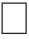2,357,799

Solution:

The numbers 2,357,822 and 2,357,799
can be placed in place value table as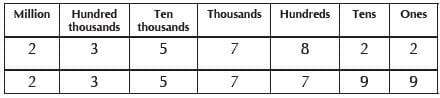It is seen that the digits at million, hundred thousands, ten thousands and thousands place are the same.

Comparing the digits at hundreds place,
8 > 7.

Thus, 2,357,822 > 2,357,799

Hence, the correct answer is B.

QUESTION: 12

The number 35 million ends with how many zeroes?

Solution:

It is known that 1 million = 1,000,000 35 million = 35,000,000

Therefore, there are six zeroes in 35
million.

Hence, the correct answer is C.

QUESTION: 13

Which one of the following numbers is prime?

Solution:

18 is composite because 18 can be written as a product of factors, not including 1 and 18 :

18 = 2×9 or 18 = 3×6.

19 is prime because 19 doesn’t have
any factors except 1 and 19.

20 is composite because 20 can be written as a product of factors, not including 1 and 20 :

20 = 2×10 or 20 = 4×5

21 is composite because 21 can be written as a product of factors, not including 1 and 21 : 21 = 3×7

QUESTION: 14

Which one of the following numbers is prime?

Solution:

26 is composite because 26 can be written as a product of factors, not including 1 and 26 :

26 = 2 × 13.

27 is composite because 27 can be written as a product of factors, not including 1 and 27:

27 = 3 × 3 × 3 or 33

28 is composite because 28 can be written as a product of factors, not including 1 and 28:

28 = 4 × 7 or 22 × 7.

29 is prime because 29 doesn’t have any factors except 1 and 29.

QUESTION: 15

Which one of the following numbers is composite?

Solution:

69 is composite number. 67, 71 and 73 are all prime numbers.

QUESTION: 16

Which one of the following numbers is composite?

Solution:

105 = 3 × 35 or 3 × 5 × 7, and so is composite.

101, 103 and 107 are all prime numbers.

QUESTION: 17

The number 24 is to be written as a product of its prime factors. Which one of the following is correct?

Solution:

2 and 3 are prime numbers; 4, 6 and 8 are composite numbers.

All the products are correct, but only answer D is a product of prime factors

QUESTION: 18

The number 90 is to be written as a product of its prime factors. Which one of the following is correct?

Solution:

2, 3 and 5 are prime numbers; 6, 9 and
15 are composite numbers.

All the products are correct, but only
answer B has a product of prime factors.

QUESTION: 19

The place value of 5 in 780756 is

Solution:

Place value of 5 is 5 × 10 = 50

QUESTION: 20

Match the following numbers in list I with the corresponding place value of number 5.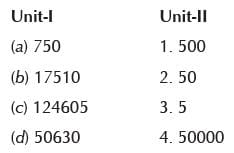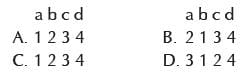Solution:

Place value of 5 in
750 = 5 × 10 = 50
17510 = 5 × 100 = 500
124605 = 5 × 1 = 5
50630 = 5 × 10000 = 50000Use Code STAYHOME200 and get INR 200 additional OFF Use Coupon Code